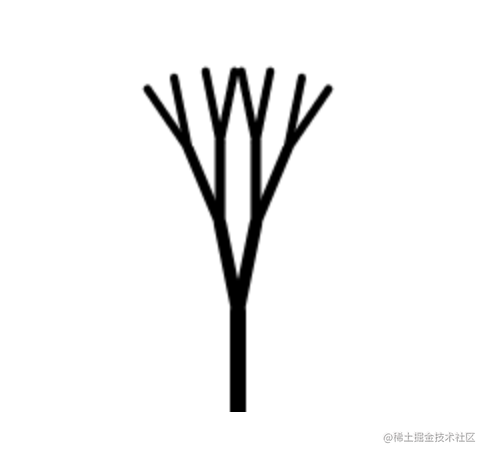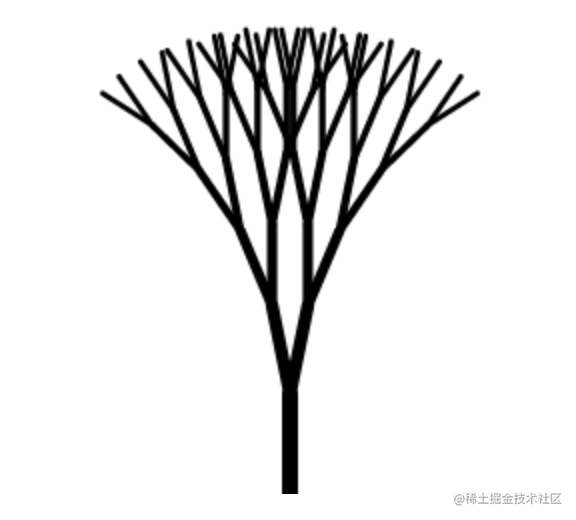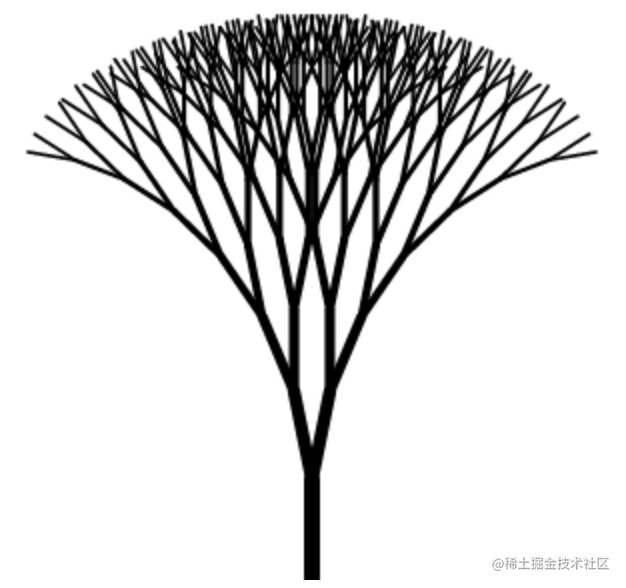# 基础数学篇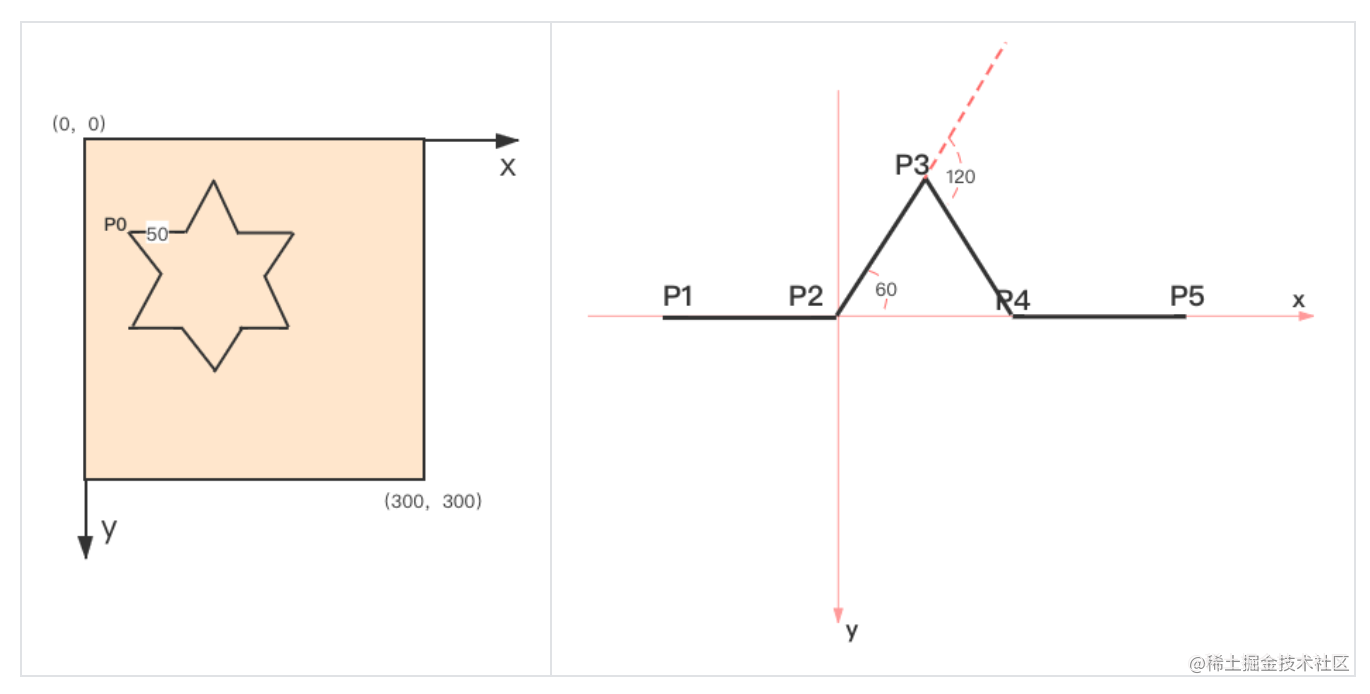## 旋转与坐标点映射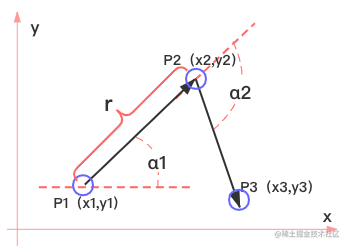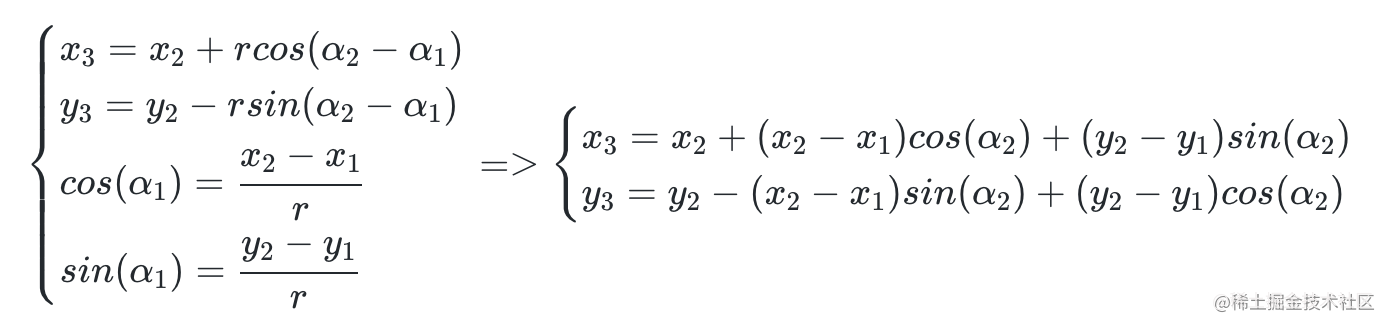同理可以得到逆时针旋转的计算公式。由以上推导同样可以得到旋转矩阵如下。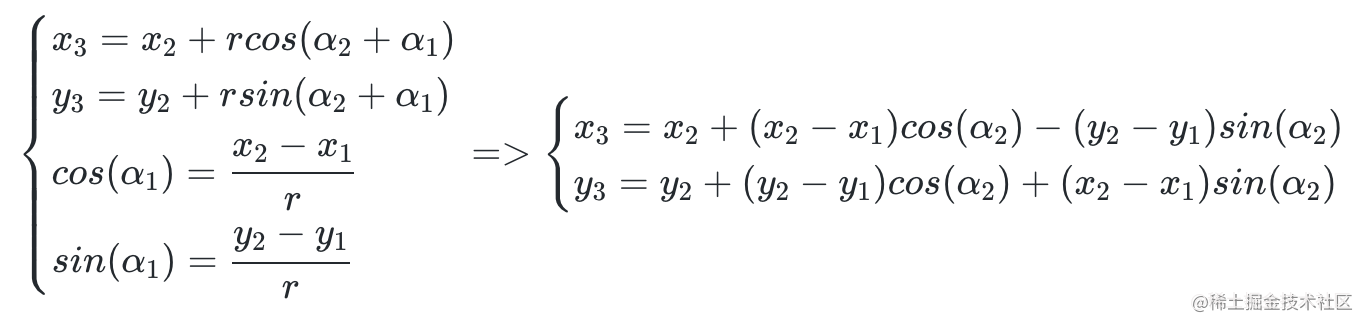### 代码实现

1.   绘制六角形。首先从第一个点P1$$0,50$$、P2$$50,50$$开始画，核心模块放入hexagon方法中，迭代6次。
复制代码
  var ctx = canvas.getContext("2d");
ctx.strikeStyle = "#000";
ctx.beginPath();
const y = 50;
ctx.moveTo(0, y);
hexagon(ctx, 0, y, 50, y, 0, 6);

1. hexagon 方法的输入参数有(ctx, x1, y1, x2, y2, n, m)。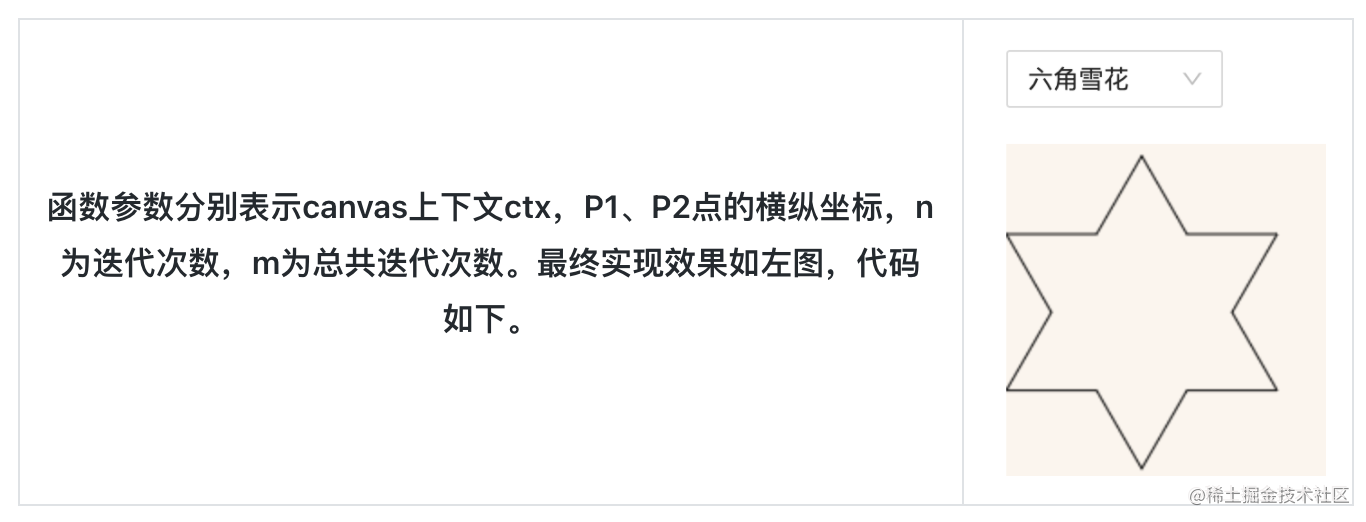function hexagon(ctx, x1, y1, x2, y2, n, m) {

ctx.clearRect(0, 0, 300, 300);

// 顺时针 60度
ctx.moveTo(x2, y2);

const x3 = x2 +

(x2 - x1) * Math.cos(Math.PI / 3) +

(y2 - y1) * Math.sin(Math.PI / 3);

const y3 = y2 -

(x2 - x1) * Math.sin(Math.PI / 3) +

(y2 - y1) * Math.cos(Math.PI / 3);

ctx.lineTo(x3, y3);

// 逆时针 120度
const x4 = x3 +

(x3 - x2) * Math.cos((Math.PI * 2) / 3) -

(y3 - y2) * Math.sin((Math.PI * 2) / 3);

const y4 = y3 +

(y3 - y2) * Math.cos((Math.PI * 2) / 3) +

(x3 - x2) * Math.sin((Math.PI * 2) / 3);

ctx.lineTo(x4, y4);

ctx.stroke();

n++;

if (n === m) {

return false;

} else {

hexagon(ctx, x3, y3, x4, y4, n, m);

}

}

## 向量运算

### 代码实现

class Vector extends Array {

constructor(x = 1, y = 0) {

super(x, y);

}

copy() {

return new Vector(this.x, this.y);

}

}

  add(v) {

this.x += v.x;

this.y += v.y;

return this;

}

sub(v) {

this.x -= v.x;

this.y -= v.y;

return this;

}

  cross(v) {

return this.x * v.y - v.x * this.y;

}

dot(v) {

return this.x * v.x + v.y * this.y;

}

  rotate(rad) {

const [x, y] = this;

this.x = x * c + y * -s;

this.y = x * s + y * c;

return this;

}

 var ctx = canvas.getContext("2d");

ctx.translate(0, canvas.height);

ctx.scale(1, -1);

const v0 = new Vector(0, 250);

const v1 = new Vector(50, 250);

hexagon(ctx, v0, v1, 0, 6);

function hexagon(ctx, v0, v1, n, m) {

const v2 = v1.copy().sub(v0);

v2.rotate((60 * Math.PI) / 180).add(v1); // 逆时针 60度

const v3 = v2.copy().sub(v1);

v3.rotate((-120 * Math.PI) / 180).add(v2); // 顺时针 120度

ctx.beginPath();

ctx.moveTo(...v1);

ctx.lineTo(...v2);

ctx.lineTo(...v3);

ctx.stroke();

n++;

if (n === m) {

return false;

} else {

hexagon(ctx, v2, v3, n, m);

}

}

# 实践篇

## 科赫雪花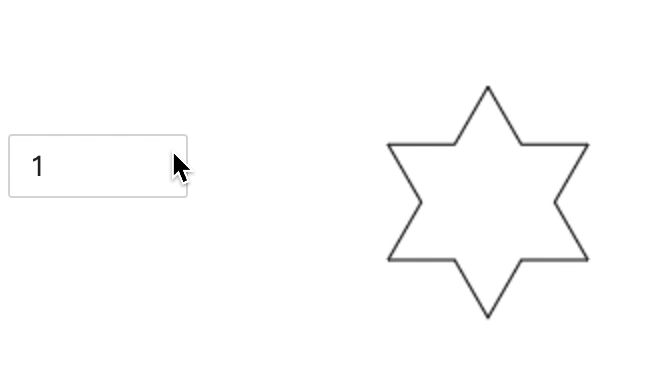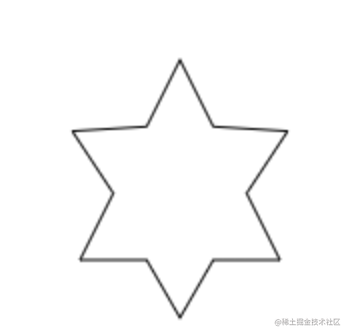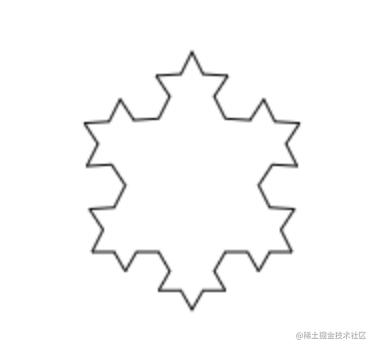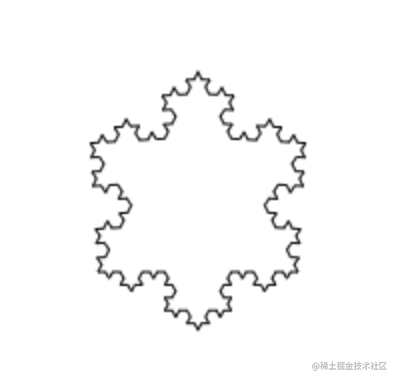### 思路

1. 科赫雪花由科赫曲线组成，它最基本的形状是一个三角形，将三角形的每条边等分成 3 份，中间那份线段先右转 60 度之后画出边长一样的线段后，再向左旋转 120 度画出等长。
2. 在递归的模块里递归执行步骤 1，直到达到设置的递归层级。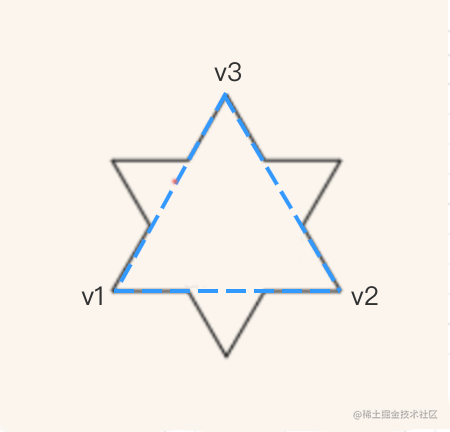图 4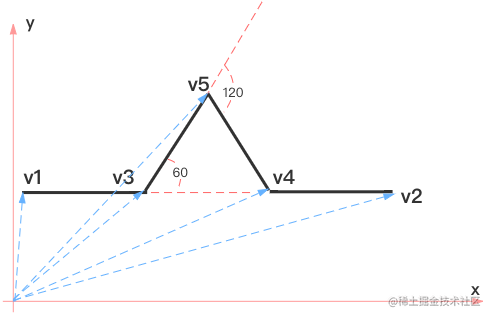图 5

### 代码实现

 var ctx = canvas.getContext("2d");

ctx.translate(0, canvas.height);

ctx.scale(1, -1);

 var ctx = canvas.getContext("2d");

const v1 = new Vector(100, 100);

const v2 = new Vector(100, 0).add(v1);

const v3 = new Vector(100, 0).rotate((60 * Math.PI) / 180).add(v1);

      koch(ctx, v1, v2, 0, deep);

koch(ctx, v2, v3, 0, deep);

koch(ctx, v3, v1, 0, deep);

  function koch(ctx, v1, v2, n, m) {

ctx.clearRect(0, 0, 300, 300); //每次绘图前清除画板

const oneThirdVector = v2
.copy()
.sub(v1)
.scale(1 / 3);

const v5 = v4
.copy()
.sub(v3)
.rotate((-60 * Math.PI) / 180)

n++;

if (n === m) {

//绘图（连线） 当前层级与设定层级一致时候停止递归

ctx.moveTo(...v1);

ctx.lineTo(...v3);

ctx.lineTo(...v5);

ctx.lineTo(...v4);

ctx.lineTo(...v2);

ctx.stroke();

return false;

}

//递归调用绘图

koch(ctx, v1, v3, n, m);

koch(ctx, v3, v5, n, m);

koch(ctx, v5, v4, n, m);

koch(ctx, v4, v2, n, m);

}

## 六角形雪花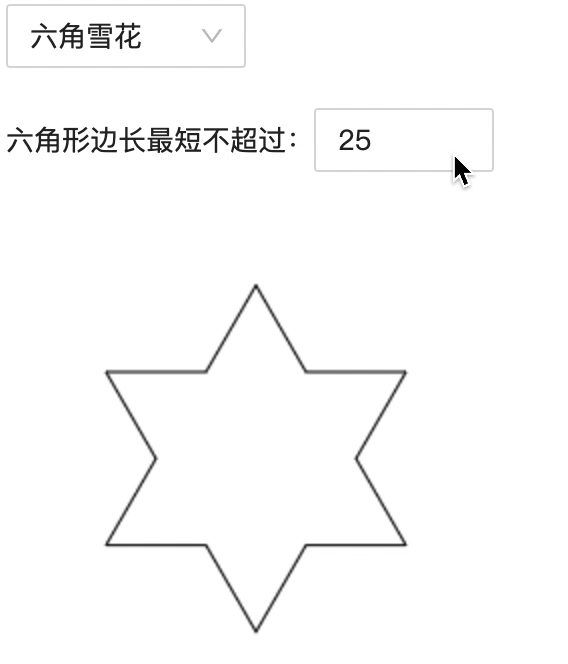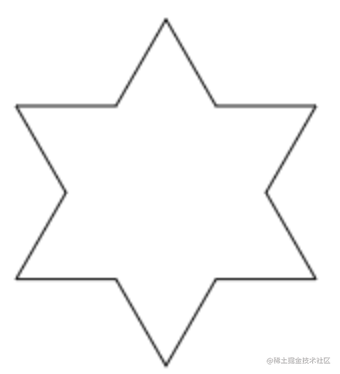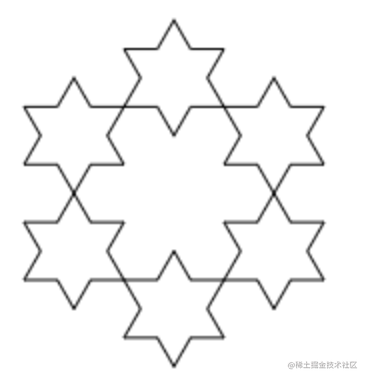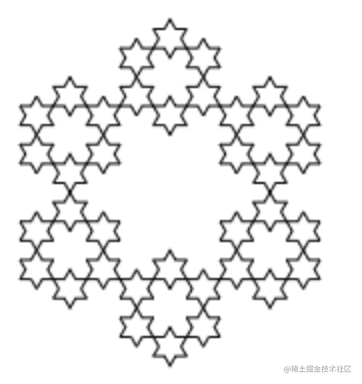### 思路

1. 首先根据基础篇中提到的六角形雪花的生成规律，每相邻两条边为一组，第 1 条边左转 60 度，第 2 条边右转 120 度，执行 6 次即可得到基础形状六角形。
2. 在开始画线之前判断该次的边长是否小于规定的最小边长。如果不小于则将边长变为原变成的 1/3，进行递归调用，若小于则开始绘制六角形。

### 代码实现

const v0 = new Vector(50, 200);

const v1 = new Vector(100, 200);

hexagon(ctx, v0, v1);

  function hexagon(ctx, v0, v1) {

const hexagonLen = v1.copy().sub(v0).vlength;

if (hexagonLen > minLine) {
// 进入迭代
}

}

// 迭代中 hexagon(ctx, v0, v1)

let vstart = v1.copy();

let vmiddle = v1.copy().sub(v0);

let vend = new Vector();

for (let i = 0; i < 6; i++) {
// 六角形的6次循环
}

  // 向量旋转

vend = vmiddle.copy().sub(vstart);

const thirdv = vend
.copy()
.sub(vmiddle)
.scale(1 / 3)

const newHexagonLen = thirdv.copy().sub(vmiddle).vlength;

if (newHexagonLen > minLine) {

// 递归

hexagon(ctx, vmiddle, thirdv);

} else {

// 绘图

ctx.beginPath();

ctx.moveTo(...vstart);

ctx.lineTo(...vmiddle);

ctx.lineTo(...vend);

ctx.stroke();

}

vstart = vend.copy();

vmiddle = vend.copy().sub(vmiddle);

## 二叉树

### 思路

1. 首先我们只需要知道初始状态时的起点以及树干的长度。
2. 在递归模块中，我们将树枝长度与宽度都削减为上一级树枝的 1/2。并且进行固定角度的左右偏移。终止条件为树枝的长度小于规定好的最小长度。这样就可以画出一颗二叉树了。